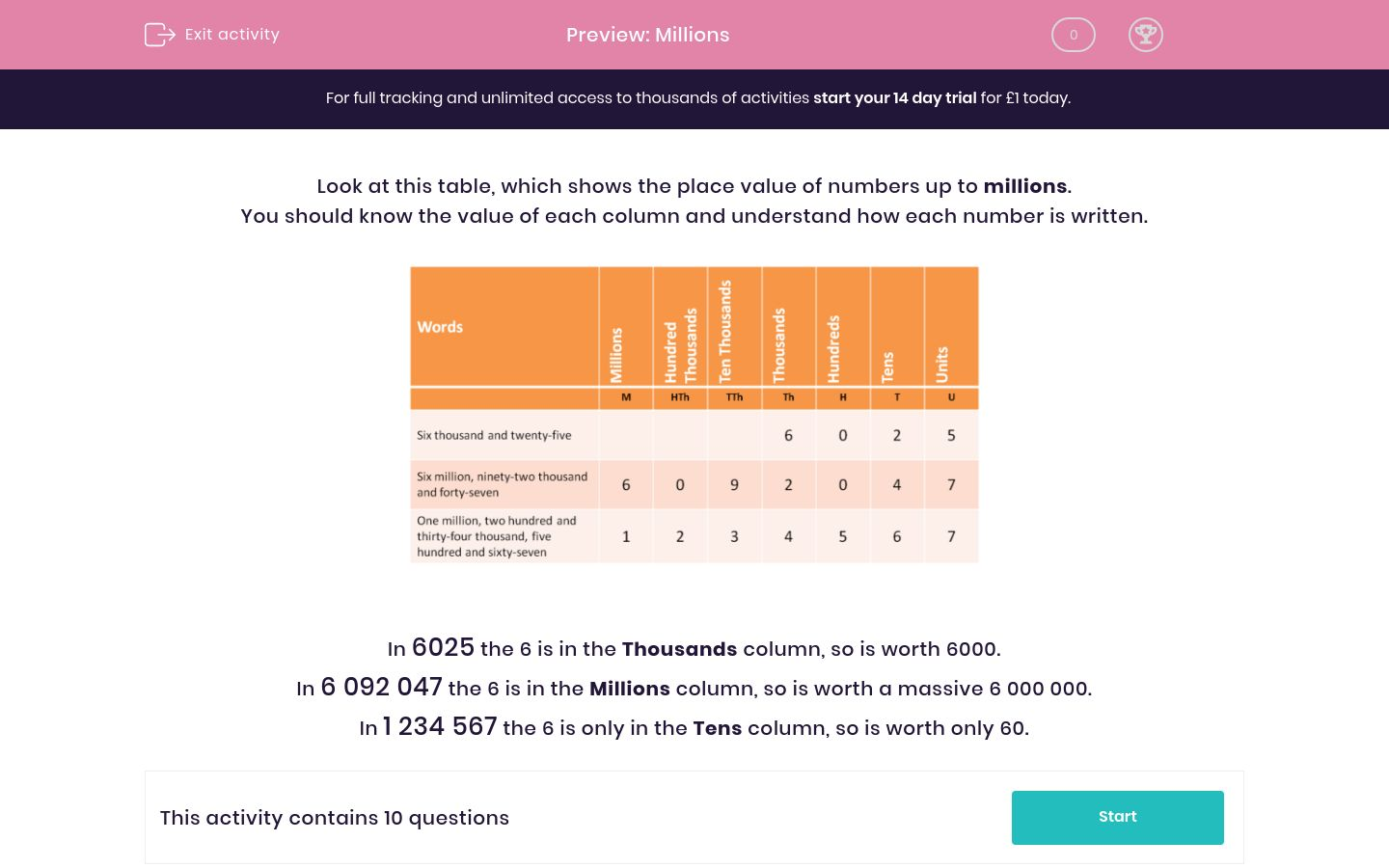# Millions

In this worksheet, students must be able to read numbers up to millions and state the value of the given digits.Key stage:  KS 2

Curriculum topic:   Maths and Numerical Reasoning

Curriculum subtopic:   Place Value

Difficulty level:### QUESTION 1 of 10

Look at this table, which shows the place value of numbers up to millions.

You should know the value of each column and understand how each number is written.In 6025 the 6 is in the Thousands column, so is worth 6000.

In 6 092 047 the 6 is in the Millions column, so is worth a massive 6 000 000.

In 1 234 567 the 6 is only in the Tens column, so is worth only 60.When you write the following number using digits, select the column in which you would place the digit  3.

Five million, three hundred and fifty-two thousand and ninety-six

Hundreds

Thousands

Ten Thousands

Hundred Thousands

Millions

Tens

UnitsWhen you write the following number using digits, select the column in which you would place the digit  3.

Three million, fifty-two thousand and ninety-six

Hundreds

Thousands

Ten Thousands

Hundred Thousands

Millions

Tens

UnitsWhen you write the following number using digits, select the column in which you would place the digit  3.

Fifty-two thousand and thirty-six

Hundreds

Thousands

Ten Thousands

Hundred Thousands

Millions

Tens

UnitsWhen you write the following number using digits, select the column in which you would place the digit  3.

Fifty-three thousand and twenty-six

Tens

Hundreds

Thousands

Ten Thousands

Hundred Thousands

Millions

UnitsWhen you write the following number using digits, select the column in which you would place the digit  3.

Five million, six hundred and fifty-three thousand, seven hundred and ninety-six

Hundreds

Thousands

Ten Thousands

Hundred Thousands

Millions

Tens

UnitsWhen you write the following number using digits, select the column in which you would place the digit  3.

Five million, six hundred and fifty-five thousand, seven hundred and ninety-three

Hundreds

Thousands

Ten Thousands

Hundred Thousands

Millions

Tens

UnitsWhen you write the following number using digits, select the column in which you would place the digit  3.

Five million, three hundred and fifty-five thousand, seven hundred and ninety

Hundreds

Thousands

Ten Thousands

Hundred Thousands

Millions

Tens

UnitsWhen you write the following number using digits, select the column in which you would place the digit  3.

Five million, eight hundred and thirty-five thousand, seven hundred and forty

Hundreds

Thousands

Ten Thousands

Hundred Thousands

Millions

Tens

UnitsWhen you write the following number using digits, select the column in which you would place the digit  3.

Five million, eight hundred thousand and thirty

Hundreds

Thousands

Ten Thousands

Hundred Thousands

Millions

Tens

UnitsWhen you write the following number using digits, select the column in which you would place the digit  3.

Five million, six hundred and fifty-two thousand, three hundred and ninety-six

Hundreds

Thousands

Ten Thousands

Hundred Thousands

Millions

Tens

Units

• Question 1When you write the following number using digits, select the column in which you would place the digit  3.

Five million, three hundred and fifty-two thousand and ninety-six

Hundred Thousands
EDDIE SAYS
3 hundred thousand.
The number is 5 352 096.
• Question 2When you write the following number using digits, select the column in which you would place the digit  3.

Three million, fifty-two thousand and ninety-six

Millions
EDDIE SAYS
3 million.
The number is 3 052 096.
• Question 3When you write the following number using digits, select the column in which you would place the digit  3.

Fifty-two thousand and thirty-six

Tens
EDDIE SAYS
Thirty is 3 tens.
The number is 52 036.
• Question 4When you write the following number using digits, select the column in which you would place the digit  3.

Fifty-three thousand and twenty-six

Thousands
EDDIE SAYS
3 thousand.
The number is 53 026.
• Question 5When you write the following number using digits, select the column in which you would place the digit  3.

Five million, six hundred and fifty-three thousand, seven hundred and ninety-six

Thousands
EDDIE SAYS
3 thousand.
The number is 5 653 796.
• Question 6When you write the following number using digits, select the column in which you would place the digit  3.

Five million, six hundred and fifty-five thousand, seven hundred and ninety-three

Units
EDDIE SAYS
Three is 3 units.
The number is 5 655 793.
• Question 7When you write the following number using digits, select the column in which you would place the digit  3.

Five million, three hundred and fifty-five thousand, seven hundred and ninety

Hundred Thousands
EDDIE SAYS
3 hundred thousand.
The number is 5 355 790.
• Question 8When you write the following number using digits, select the column in which you would place the digit  3.

Five million, eight hundred and thirty-five thousand, seven hundred and forty

Ten Thousands
EDDIE SAYS
Thirty thousand is 3 in the Ten Thousands column.
The number is 5 835 740.
• Question 9When you write the following number using digits, select the column in which you would place the digit  3.

Five million, eight hundred thousand and thirty

Tens
EDDIE SAYS
Thirty is 3 tens.
The number is 5 800 030.
• Question 10When you write the following number using digits, select the column in which you would place the digit  3.

Five million, six hundred and fifty-two thousand, three hundred and ninety-six

Hundreds
EDDIE SAYS
3 hundred.
The number is 5 652 396.
---- OR ----

Sign up for a £1 trial so you can track and measure your child's progress on this activity.

### What is EdPlace?

We're your National Curriculum aligned online education content provider helping each child succeed in English, maths and science from year 1 to GCSE. With an EdPlace account you’ll be able to track and measure progress, helping each child achieve their best. We build confidence and attainment by personalising each child’s learning at a level that suits them.

Get started